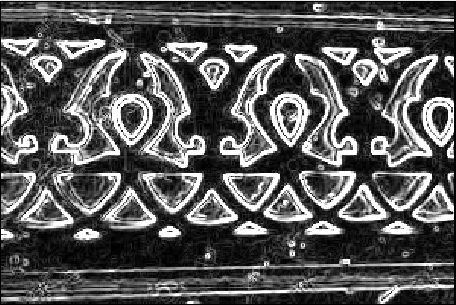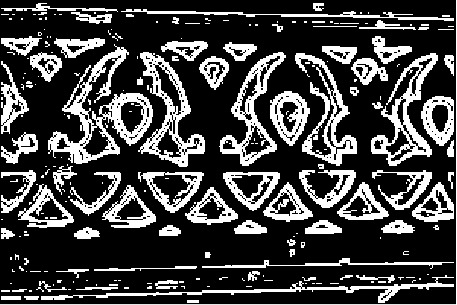Related Articles

# MATLAB – Image Edge Detection using Sobel Operator from Scratch

• Last Updated : 17 May, 2020

Sobel Operator: It is a discrete differentiation gradient-based operator. It computes the gradient approximation of image intensity function for image edge detection. At the pixels of an image, the Sobel operator produces either the normal to a vector or the corresponding gradient vector. It uses two 3 x 3 kernels or masks which are convolved with the input image to calculate the vertical and horizontal derivative approximations respectively –Approach:

Step 1: Input – Read an image
Step 2: Convert the true-color RGB image to the grayscale image
Step 3: Convert the image to double
Step 4: Pre-allocate the filtered_image matrix with zeros
Step 5: Define Sobel Operator Mask
Step 6: Edge Detection Process (Compute Gradient approximation and magnitude of vector)
Step 7: Display the filtered image
Step 8: Thresholding on the filtered image
Step 9: Display the edge-detected image

Implementation in MATLAB:

 % MATLAB Code | Sobel Operator from Scratch  % Read Input Imageinput_image = imread('[name of input image file].[file format]');  % Displaying Input Imageinput_image = uint8(input_image);figure, imshow(input_image); title('Input Image');  % Convert the truecolor RGB image to the grayscale imageinput_image = rgb2gray(input_image);  % Convert the image to doubleinput_image = double(input_image);  % Pre-allocate the filtered_image matrix with zerosfiltered_image = zeros(size(input_image));  % Sobel Operator MaskMx = [-1 0 1; -2 0 2; -1 0 1];My = [-1 -2 -1; 0 0 0; 1 2 1];  % Edge Detection Process% When i = 1 and j = 1, then filtered_image pixel  % position will be filtered_image(2, 2)% The mask is of 3x3, so we need to traverse % to filtered_image(size(input_image, 1) - 2%, size(input_image, 2) - 2)% Thus we are not considering the borders.for i = 1:size(input_image, 1) - 2    for j = 1:size(input_image, 2) - 2          % Gradient approximations        Gx = sum(sum(Mx.*input_image(i:i+2, j:j+2)));        Gy = sum(sum(My.*input_image(i:i+2, j:j+2)));                         % Calculate magnitude of vector        filtered_image(i+1, j+1) = sqrt(Gx.^2 + Gy.^2);             endend  % Displaying Filtered Imagefiltered_image = uint8(filtered_image);figure, imshow(filtered_image); title('Filtered Image');  % Define a threshold valuethresholdValue = 100; % varies between [0 255]output_image = max(filtered_image, thresholdValue);output_image(output_image == round(thresholdValue)) = 0;  % Displaying Output Imageoutput_image = im2bw(output_image);figure, imshow(output_image); title('Edge Detected Image');

Input Image –Filtered Image:Edge Detected Image:1. Simple and time efficient computation
2. Very easy at searching for smooth edges

Limitations:

1. Diagonal direction points are not preserved always
2. Sensitive to noise
3. Not very accurate in edge detection
4. Detect with thick and rough edges does not give appropriate results

My Personal Notes arrow_drop_up